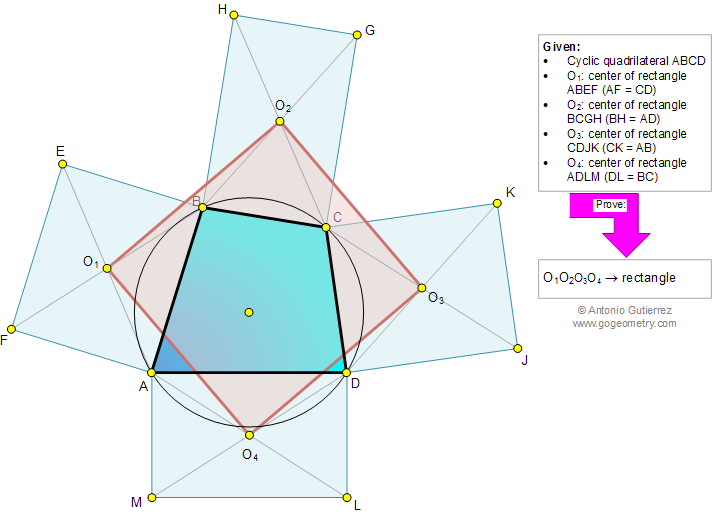# Geometry Problem 868: Cyclic Quadrilateral, Circle, Five Rectangles, Four Centers, Congruence. Level: High School, College, Mathematics Education

 The figure below shows a cyclic quadrilateral ABCD. ABEF, BCGH, CDJK, and ADLM are rectangles of centers O1, O2, O3, and O4, respectively, so that AF = CD, BH = AD, CK = AB, and DL = BC. Prove that O1O2O3O4 is a rectangle.See also: Typography of problem 868.
 Home | Search | Geometry | Problems | All Problems | Open Problems | Visual Index | 10 Problems | 861-870 | Cyclic Quadrilateral | Circle | Rectangle | Congruence | Perpendicular lines, 90 degrees | Email | Solution / comment